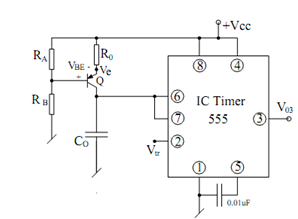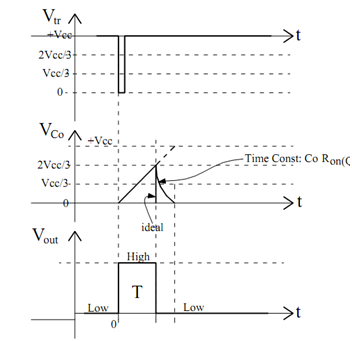## Sawtooth Generation using Monostable Multivibrator Assignment Help

Assignment Help: >> IC Timer - Sawtooth Generation using Monostable Multivibrator

Sawtooth Generation using Monostable Multivibrator:

A modified form of the basic monostable multivibrator is illustrated in Figure where the capacitor C0 charges linearly through the current source comprising of the transistor R0 - RA - RB combination.Figure: A Modified Form of Monostable Circuit for Sawtooth Generation

The several waveforms exploring the operation of this circuit are illustrated in FigureFigure: Various Waveforms for the Monostable Multivibrator

I0  = IC  ≅ I E (( V cc - V e )/ R0

Neglecting base current, we may write

V BE      = V2  -(            R B /( RA - RB ) V cc

V e  = V BE + RB /RA + RB) Vcc

Since    I0   = V cc - Ve /R0  = 1/R0  - { Vcc  -{VBE  + ( RB/RA+RB)Vcc )

I0  = { (V cc ( RA /RA+RB  )- VBE /R0)]

Since Q = C0 Vc, we may write

dQ/ dt = C0  ,    dv/ dt

I0 / C0  = dVc/ dt

If T = time taken by the capacitor to charge to a voltage2/3 Vcc, this is found to be

T =   {(2/3) Vcc  -0}/ dVc /dt =2Vcc C0 /3I0

T =       ((2/3) 2 C0   R0 V cc  )/  { Vcc RA / (RA  + RB )- V BE